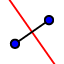# Perpendicular Bisectors of a Triangle

## Intermediate Construction 4

We are now going to construct the perpendicular bisectors of the triangle. As with the two previous examples, every triangle has three of these lines. We already learned how to use theperpendicular bisector tool to draw these lines so use this tool now to draw the three lines on the triangle below. If you need help, use the video but try to complete this on your own.

What do you notice about the three perpendicular bisectors? What happens if you change the triangle into an acute triangle? Right triangle? Obtuse triangle? Draw a circle with its center at the point you have created and the edge at point A, what do you notice?